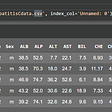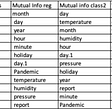# Descriptive Statistics Part 1

Section 1 -

How to Summarizing Categorical variable or Qualitative Variable.

Table of content.

1. Frequency Table.
2. bar charts.
3. Pie Chart.

To understand the concept , suppose we take a example of wines. Sample of size 200 and we have recorded of never drink peoples , past drink peoples , present drink peoples .best way to summarized the qualitative data or categorical data in frequency relative frequency is called proportion and percentage.

Let take doing that , summarizing categorical variable.

1- Frequency table , some time is called frequency distribution .[Sample Size =200]

Drinking Status

Let us Suppose 1100 is never drink , 500 is Past and 400 is present out of 2000 peoples and then this frequency convert into proportion ( relative frequency and percentage also)

So , This table is here show the distribution.

Note- Larger sample size good for is meaning report of proportion and percentage and if have smaller sample sizes , suppose we have only 200 individual. 110 falling in never drink , 50 is past and 40 is present then frequency will meaningful rather than proportion and percentage.

If we make a plot and visualize table rather than numbers. if table is larger and bigger so, not easy to understand table in numeric form. so , then we draw bar chat or pie chart of this table.

2- Bar chart

We take on x-axis one of these frequency proportions and percent , so we choose proportion.

This bar separate and space between them. It indicate these are separate categories , there no continuity between the two so this chart help to distribute for the variable.

3- Pie Chart

One other plot we can make for this table is categorized table that is pie chart.

It deal percentage of table to show distribution in each categorized.

It is pie , this circle represent entire sample and each level and categorized we draw in silence in pie.

This is another way of showing and visualizing categorical variable.

Section 2-

How to Summarizing Numeric variable or Quantitative Variable.

1- Frequency Table.

2. Histogram.

3. Kernel Density Plot.

Understand what is and Why it useful.

We take sample example , we Collect same of size is 50 and recorded the ages for a bunch of individuals.

so, histogram and density plots display the ( — -)distribution of a sample for a numeric (quantitative / continuous)variable.

These plot help to visualize the distribution of our sample for a numerical quantitative data.

Age = 50

4,6,7,4,12,13,16,19,22………..

1- Frequency table.

Note – This when useful we have bunches of data and no. again & again repeat so, Its easy way to distribute the data.

One another point , if you change interval these frequency change slightly.

So , We can draw a picture or a plot.

Q-Why plot and picture need?

Ans- Because look at table is look messy to look so, we draw the plot and picture for easy understand of messy table and data.

Now for continuous numeric data we create histogram-

2- Histogram.

Its nice visual to show the distribution , how the people distributed for the variable age.

Note- notice that each bars are touching that’s because age is this continuous measurement there’s no space between bars.

In bar charts each categorized is separate but in histogram each categorized continuous no space between them.

Note- If any change in bins(intervals) mean 0–20 , 20–40, bigger, so shape of graph might change slightly .

Note 1- Its important to note that this graph help us to visualize a lot about the variable age , we can kind of see, what is the center. where 50% data which range , what is highest mean etc. we will discuss later.

3- Kernel density plots

Q- Without use mathematics and technical thing , what it is ?

Ans- Its sort of a smoothing out of this histogram here and try to get a idea that mentioned if we change the bing ( intervals) slightly of the shape of this us going to change a little bit.

So ,kernel density plot is smoothness version of histogram. It tell smoothness of the histogram.

So, these two plots are useful to summarize or visualize the sample numeric or continuous variable of given us a sort of estimate of what probability distribution.

4- Box plot

We use box plot for finding five keys.box plot make using 5 keys value.

• Median.
• First quartile value.
• Third quartile value.
• Min value
• Max value

Most of the time or mostly box plot use for continuous data for finding above 5 keys.

How Analysis more precisely about continuous data using Box plot go to second part.

If any doubt regarding it. please comment below and ask feel free.

--

--

--

## More from Puneet Singh

Data Science , Machine Learning , BlockChain Developer

Love podcasts or audiobooks? Learn on the go with our new app.

## Using Python’s Scikit-Learn(Sklearn) for Data Science## Taking Your First Data Science Project From Start to Finish## Ranking MMA fighters Part 2: The Glicko rating system## Predicting Hepatitis## Puneet Singh

Data Science , Machine Learning , BlockChain Developer

## Learning machine learning for Senior Management — You Just Have to Jump in Systematically## Combating human trafficking using machine learning: Part 4.## A magical day in Disney with Machine Learning — Part 8 Feature Selection Discrimination